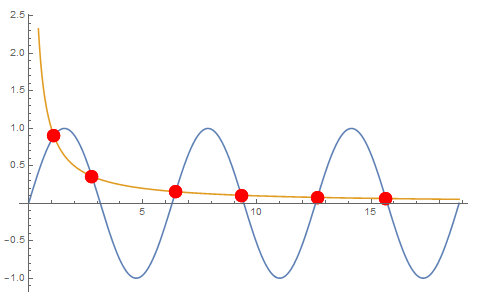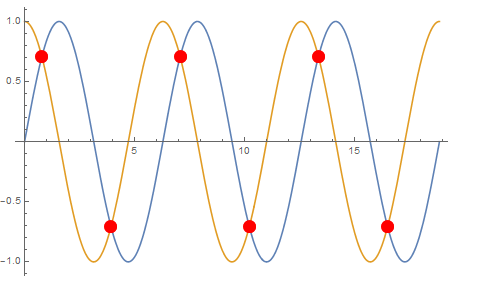# Mathematica画两函数交点图

• A+

## 代码实现

• 这一个是函数Sin[x]和函数x在范围0到6Pi之间的交点。
``````Plot[{Sin[x], 1/x}, {x, 0, 6 Pi}, Mesh -> {{0.}},
MeshFunctions -> Function[x, 1/x - Sin[x]],
MeshStyle -> Directive[PointSize[0.03], Red]
]``````• 这一个是函数Sin[x]和函数Cos[x]在范围0到6Pi之间的交点。
``````Plot[{Sin[x], Cos[x]}, {x, 0, 6 Pi}, Mesh -> {{0.}},
MeshFunctions -> {Sin[#] - Cos[#] &},
MeshStyle -> Directive[PointSize[0.03], Red]
]``````• 微信公众号
• 关注微信公众号
•• QQ群
• 我们的QQ群号
•